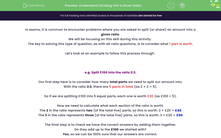# Understand Dividing into a Given Ratio

In this worksheet, students will split amounts into given ratios using the method of finding the total number of parts, dividing by this value, and then multiplying by each value in the ratio.Key stage:  KS 4

Year:  GCSE

GCSE Subjects:   Maths

GCSE Boards:   AQA, Eduqas, Pearson Edexcel, OCR,

Curriculum topic:   Ratio, Proportion and Rates of Change

Curriculum subtopic:   Ratio, Proportion and Rates of Change Calculations with Ratio

Difficulty level:#### Worksheet Overview

In exams, it is common to encounter problems where you are asked to split (or share) an amount into a given ratio.

We will be focusing on this skill during this activity.

The key to solving this type of question, as with all ratio questions, is to consider what 1 part is worth.

Let's look at an example to follow this process through.

e.g. Split £100 into the ratio 2:3.

Our first step here is to consider how many total parts we need to split our amount into.

With the ratio 2:3, there are 5 parts in total (as 2 + 3 = 5).

So if we are splitting £100 into 5 equal parts, each one is worth £20 (as £100 ÷ 5).

Now we need to calculate what each section of the ratio is worth.

The 2 in the ratio represents two (of the total five) parts, so this is worth: 2 × £20 = £40

The 3 in the ratio represents three (of the total five) parts, so this is worth: 3 × £20 = £60

The final step is to check we have the correct answers by adding them together.

Do they add up to the £100 we started with?

Yes, so we can be 100% sure that our answers are correct.

In this activity, we will split amounts into given ratios using the method demonstrated above of finding the total number of parts, dividing by this value, and then multiplying by each value in the ratio.

### What is EdPlace?

We're your National Curriculum aligned online education content provider helping each child succeed in English, maths and science from year 1 to GCSE. With an EdPlace account you’ll be able to track and measure progress, helping each child achieve their best. We build confidence and attainment by personalising each child’s learning at a level that suits them.

Get started